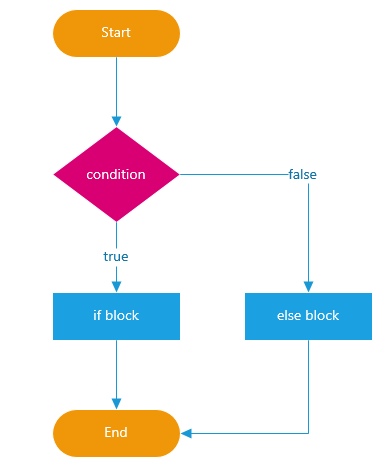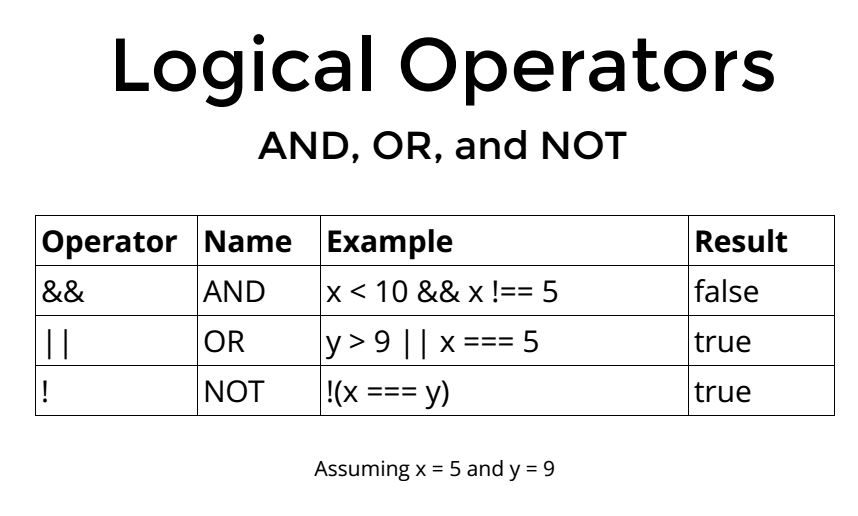# p5: conditionals / if statementsA condition like `if( myVariable == true)` or` if(myVariable > 20)` must always return a boolean value (true or false).

To construct more complex conditions for both if statements and loops we have to get into Boolean operators.For our purposes == and === are equivalent. In javascript they compare both value and type of a variable.

### Exercise

Starting from  a 9×9 pattern of green circles

```var rows = 9;
var rows = 9;
var cols = 9;
var circleDiameter = 40;
var distanceX = 60;
var distanceY = 60;

function setup() {
createCanvas(600, 600);
noStroke();
}

function draw() {
background(0);
for (var r = 0; r < rows; r++) {
for (var c = 0; c < cols; c++) {

if (r == 0) {
fill(200, 0, 0);
} else {
fill(0, 200, 0);
}

ellipse(c * distanceX + distanceX, r * distanceY + distanceY, circleDiameter, circleDiameter);
}
}
}```

Reproduce the following outputs by changing only the if condition: